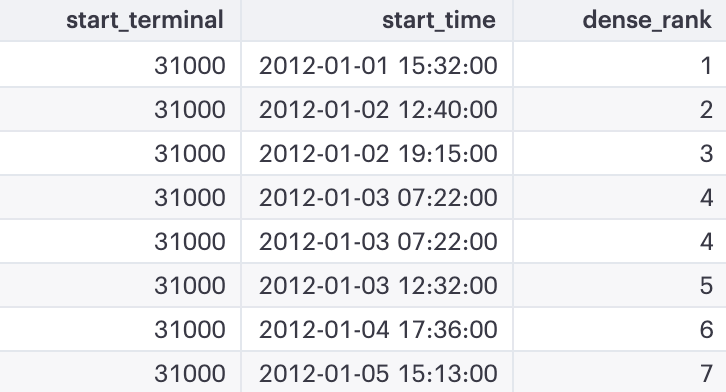# SQL Advanced Cheatsheet — Window Functions

Window functions are similar to aggregate functions in that they compute aggregate values by performing calculations across a set of rows related to the current row. Unlike aggregate functions, using window functions does not group the result set (into a single output row) but instead retains the same number of rows as the input.

Does this sound confusing? Let’s dissect window functions by going over the basic structures and a few examples.

# Window Function Basics

`SELECT duration,        SUM(duration) OVER (ORDER BY start_time) AS running_totalFROM bikeshare_stats`

The above query sums duration seconds over the entire result set, in order by start time. Let’s look at the basic syntax of window functions:

Suppose we’d like to narrow the window function from the whole dataset to individual groups, we can use PARTITION BY, which is similar to GROUP BY:

`SELECT start_terminal, duration,        SUM(duration) OVER (PARTITION BY start_terminal                            ORDER BY start_time) AS running_totalFROM bikeshare_stats`

Now, the query is grouped by start_terminal and within each start_terminal value, it is ordered by start_time.

Notice that running_total (summing duration seconds) starts over when we reach a new start_terminal. This happens when we group using PARTITION BY — all aggregate functions are performed within individual groups. In addition, ORDER BY treats every partition separately.

Essentially, ORDER BY and PARTITION BY defines the “window” — the ordered subset of data in which aggregate calculations are made.

# ROW_NUMBER

ROW_NUMBER() returns the number of a given row. Suppose we’re interested in ranking the highest-paid employees in each department.

`SELECT *, ROW_NUMBER() OVER (PARTITION BY department_ID                             ORDER BY salary DESC) AS rank_in_depFROM employee_salaries`

# RANK and DENSE_RANK

We can also use RANK() or DENSE_RANK() to display rankings within individual groups. Unlike ROW_NUMBER(), both RANK() and DENSE_RANK() will display identical rankings in the case of ties. Let’s return to the earlier bike share example. Suppose we want to return the start time rankings (in ascending chronological order) within each start terminal:

`SELECT start_terminal, duration,        RANK() OVER (PARTITION BY start_terminal                     ORDER BY start_time) AS rankFROM bikeshare_stats`

We will use ROW_NUMBER(), RANK(), and DENSE_RANK() in the above query to compare the differences:Rows 4 and 5 have identical start_time

In this case, the 4th and 5th rows have the same value. ROW_NUMBER() assigns a distinct value to each row, while the other two give the identical rows the same rank. However, RANK() will skip the duplicate rank (#5 in this case is skipped due to a tie for #4) while DENSE_RANK() will not skip any ranks.

# LAG and LEAD

LAG() pulls from previous rows and LEAD() pulls from the following rows. This will be especially useful if you want to compute the differences between rows.

`SELECT start_terminal, duration,        LAG(duration, 1) OVER (PARTITION BY start_terminal                              ORDER BY duration) AS lag,        LEAD(duration, 1) OVER (PARTITION BY start_terminal                               ORDER BY duration) AS lead,        duration - LAG(duration, 1) OVER (PARTITION BY start_terminal                                   ORDER BY duration) AS differenceFROM bikeshare_stats`

# NTILE

NTILE() will output what percentile a given row falls under (more specifically NTILE(100)). You could also specify quartile using NTILE(4).

Suppose we wanted to find each user’s email activity percentile, which is defined by the total number of emails sent:

`SELECT user, COUNT(*) AS total_emails,        NTILE(100) OVER (ORDER BY COUNT(*) DESC) AS email_percentileFROM gmail_statsGROUP BY user`

M.S.E Data Science @Johns Hopkins University with a B.S. in Applied Mathematics. Previously @MongoDB, current Data Science Intern @EA

## More from Alexander Chang

M.S.E Data Science @Johns Hopkins University with a B.S. in Applied Mathematics. Previously @MongoDB, current Data Science Intern @EA